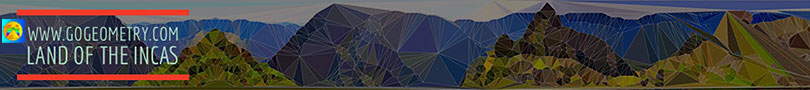Problem 113. Area of Triangles, Incircle, Excircles. Level: High School, SAT Prep, College
 In the figure below, given a triangle ABC, construct the incircle with incenter I and excircles with excenters E1, E2, and E3. Let be D, E, F, G, H, and M the tangent points of triangle ABC with its excircles. If S1, S'1, S2, S'2, S3, and S'3 are the areas of the shaded triangles, prove that S1 = S'1, similarly S2 = S'2, and S3 = S'3. View or post a solution..
 Home | Search | Problems | 111-120 | Circle Tangent Line | Email | By Antonio Gutierrez